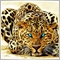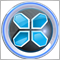# Opening orders 2 or 3 bars after MA cross80

Guys i need help with the following...

I am trying to open a buy order 2 or 3 bars after fast ma crosses slow ma and when the rsi is greater than 50 as well as when both stochastic lines are greater than 50.

A sell order for the opposite. The code below is an attempt at a buy order for the 1 minute period, however, it doesn't open any orders.

```if(Period()==1)
{
{
if(getM1_MA(FastMAPeriod,1)>getM1_MA(SlowMAPeriod,1))
{
if(getM1_MA(FastMAPeriod,2)>getM1_MA(SlowMAPeriod,2))
{
if((getM1_MA(FastMAPeriod,1)-getM1_MA(SlowMAPeriod,1))>MathAbs(UsePoint))
{
if(M1_FastStoc>50)
{
if(M1_SlowStoc>50)
{
if(M1_RSI>50)
{
if(getM1_MA(FastMAPeriod,3)<getM1_MA(SlowMAPeriod,3)
|| (getM1_MA(FastMAPeriod,4)<getM1_MA(SlowMAPeriod,4)))
{
if(OrderSelect(M1_SellTicket,SELECT_BY_TICKET))
{
if(OrderCloseTime()==0)
{
if(M1_SellTicket>0)
{
double M1_CloseLots=OrderLots();
bool M1_Closed=OrderClose(M1_SellTicket,M1_CloseLots,
M1_ClosePrice,UseSlippage,Red);
if(M1_Closed)
{
}
}
}
}
// Calculate stop loss and take profit
M1_SellTicket=0;
}
}
}
}
}
}
}
}

double getM1_MA(int bar_period,int bar_index)
{
return iMA(Symbol(),PERIOD_M1,bar_period,0,MODE_EMA,PRICE_CLOSE,bar_index);
}```31054

Hi,

I checked it on my side it's working,So you would get some stochastic and RSI level values down(Decrease the level values),

Regards.80

Hi,

I checked it on my side it's working,So you would get some stochastic and RSI level values down(Decrease the level values),

Regards.

You are right, it's working. Thanks for your help.

Kind Regards31054

Malaza7557: